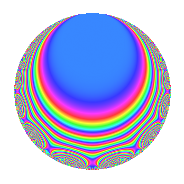# Properties

 Label 1575.1.eLevel 1575 Weight 1 Character orbit e Rep. character $$\chi_{1575}(874,\cdot)$$ Character field $$\Q$$ Dimension 8 Newform subspaces 3 Sturm bound 240 Trace bound 4

# Related objects

## Defining parameters

 Level: $$N$$ $$=$$ $$1575 = 3^{2} \cdot 5^{2} \cdot 7$$ Weight: $$k$$ $$=$$ $$1$$ Character orbit: $$[\chi]$$ $$=$$ 1575.e (of order $$2$$ and degree $$1$$) Character conductor: $$\operatorname{cond}(\chi)$$ $$=$$ $$35$$ Character field: $$\Q$$ Newform subspaces: $$3$$ Sturm bound: $$240$$ Trace bound: $$4$$

## Dimensions

The following table gives the dimensions of various subspaces of $$M_{1}(1575, [\chi])$$.

Total New Old
Modular forms 38 10 28
Cusp forms 14 8 6
Eisenstein series 24 2 22

The following table gives the dimensions of subspaces with specified projective image type.

$$D_n$$ $$A_4$$ $$S_4$$ $$A_5$$
Dimension 8 0 0 0

## Trace form

 $$8q - 6q^{4} + O(q^{10})$$ $$8q - 6q^{4} + 2q^{11} - 2q^{14} + 4q^{16} - 2q^{29} - 10q^{46} - 8q^{49} - 2q^{56} + 4q^{64} + 2q^{71} + 2q^{74} + 2q^{79} - 2q^{86} + O(q^{100})$$

## Decomposition of $$S_{1}^{\mathrm{new}}(1575, [\chi])$$ into newform subspaces

Label Dim. $$A$$ Field Image CM RM Traces $q$-expansion
$$a_2$$ $$a_3$$ $$a_5$$ $$a_7$$
1575.1.e.a $$2$$ $$0.786$$ $$\Q(\sqrt{-1})$$ $$D_{3}$$ $$\Q(\sqrt{-7})$$ None $$0$$ $$0$$ $$0$$ $$0$$ $$q-iq^{2}-iq^{7}-iq^{8}+q^{11}-q^{14}+\cdots$$
1575.1.e.b $$2$$ $$0.786$$ $$\Q(\sqrt{-1})$$ $$D_{2}$$ $$\Q(\sqrt{-3})$$, $$\Q(\sqrt{-7})$$ $$\Q(\sqrt{21})$$ $$0$$ $$0$$ $$0$$ $$0$$ $$q+q^{4}-iq^{7}+q^{16}-iq^{28}-iq^{37}+\cdots$$
1575.1.e.c $$4$$ $$0.786$$ $$\Q(\zeta_{12})$$ $$D_{6}$$ $$\Q(\sqrt{-7})$$ None $$0$$ $$0$$ $$0$$ $$0$$ $$q+(-\zeta_{12}^{2}-\zeta_{12}^{4})q^{2}+(-1-\zeta_{12}^{2}+\cdots)q^{4}+\cdots$$

## Decomposition of $$S_{1}^{\mathrm{old}}(1575, [\chi])$$ into lower level spaces

$$S_{1}^{\mathrm{old}}(1575, [\chi]) \cong$$ $$S_{1}^{\mathrm{new}}(175, [\chi])$$$$^{\oplus 3}$$

## Hecke characteristic polynomials

$p$ $F_p(T)$
$2$ ($$1 - T^{2} + T^{4}$$)($$( 1 - T )^{2}( 1 + T )^{2}$$)($$( 1 - T + T^{2} )^{2}( 1 + T + T^{2} )^{2}$$)
$3$ 1
$5$ 1
$7$ ($$1 + T^{2}$$)($$1 + T^{2}$$)($$( 1 + T^{2} )^{2}$$)
$11$ ($$( 1 - T + T^{2} )^{2}$$)($$( 1 + T^{2} )^{2}$$)($$( 1 - T^{2} + T^{4} )^{2}$$)
$13$ ($$( 1 + T^{2} )^{2}$$)($$( 1 + T^{2} )^{2}$$)($$( 1 + T^{2} )^{4}$$)
$17$ ($$( 1 + T^{2} )^{2}$$)($$( 1 + T^{2} )^{2}$$)($$( 1 + T^{2} )^{4}$$)
$19$ ($$( 1 - T )^{2}( 1 + T )^{2}$$)($$( 1 - T )^{2}( 1 + T )^{2}$$)($$( 1 - T )^{4}( 1 + T )^{4}$$)
$23$ ($$1 - T^{2} + T^{4}$$)($$( 1 - T )^{2}( 1 + T )^{2}$$)($$( 1 - T + T^{2} )^{2}( 1 + T + T^{2} )^{2}$$)
$29$ ($$( 1 + T + T^{2} )^{2}$$)($$( 1 + T^{2} )^{2}$$)($$( 1 - T^{2} + T^{4} )^{2}$$)
$31$ ($$( 1 - T )^{2}( 1 + T )^{2}$$)($$( 1 - T )^{2}( 1 + T )^{2}$$)($$( 1 - T )^{4}( 1 + T )^{4}$$)
$37$ ($$1 - T^{2} + T^{4}$$)($$( 1 + T^{2} )^{2}$$)($$( 1 - T^{2} + T^{4} )^{2}$$)
$41$ ($$( 1 - T )^{2}( 1 + T )^{2}$$)($$( 1 - T )^{2}( 1 + T )^{2}$$)($$( 1 - T )^{4}( 1 + T )^{4}$$)
$43$ ($$1 - T^{2} + T^{4}$$)($$( 1 + T^{2} )^{2}$$)($$( 1 - T^{2} + T^{4} )^{2}$$)
$47$ ($$( 1 + T^{2} )^{2}$$)($$( 1 + T^{2} )^{2}$$)($$( 1 + T^{2} )^{4}$$)
$53$ ($$( 1 + T^{2} )^{2}$$)($$( 1 - T )^{2}( 1 + T )^{2}$$)($$( 1 - T )^{4}( 1 + T )^{4}$$)
$59$ ($$( 1 - T )^{2}( 1 + T )^{2}$$)($$( 1 - T )^{2}( 1 + T )^{2}$$)($$( 1 - T )^{4}( 1 + T )^{4}$$)
$61$ ($$( 1 - T )^{2}( 1 + T )^{2}$$)($$( 1 - T )^{2}( 1 + T )^{2}$$)($$( 1 - T )^{4}( 1 + T )^{4}$$)
$67$ ($$1 - T^{2} + T^{4}$$)($$( 1 + T^{2} )^{2}$$)($$( 1 - T^{2} + T^{4} )^{2}$$)
$71$ ($$( 1 - T + T^{2} )^{2}$$)($$( 1 + T^{2} )^{2}$$)($$( 1 - T^{2} + T^{4} )^{2}$$)
$73$ ($$( 1 + T^{2} )^{2}$$)($$( 1 + T^{2} )^{2}$$)($$( 1 + T^{2} )^{4}$$)
$79$ ($$( 1 - T + T^{2} )^{2}$$)($$( 1 + T )^{4}$$)($$( 1 - T + T^{2} )^{4}$$)
$83$ ($$( 1 + T^{2} )^{2}$$)($$( 1 + T^{2} )^{2}$$)($$( 1 + T^{2} )^{4}$$)
$89$ ($$( 1 - T )^{2}( 1 + T )^{2}$$)($$( 1 - T )^{2}( 1 + T )^{2}$$)($$( 1 - T )^{4}( 1 + T )^{4}$$)
$97$ ($$( 1 + T^{2} )^{2}$$)($$( 1 + T^{2} )^{2}$$)($$( 1 + T^{2} )^{4}$$)